# Chapter 2.1. Simple Calculations

In this chapter we are going to get familiar with the following concepts and programming techniques:

• How to work with data types and variables which we need when processing numbers and strings.
• How to print a result on the screen.
• How to read a custom input.
• How to do simple arithmetic operations: addition, subtraction, multiplication, division, concatenate strings.
• How to round numbers.

## Video

Watch a video tutorial based on this chapter here: https://www.youtube.com/watch?v=kP_1cKnciyA.

## Calculations in Programming

We know that computers are machines that process data. All data is stored in the computer memory (RAM) in variables. Variables are named memory areas that store data of a certain type, for example, number or string. Each variable in JavaScript has a name and value. Here is how we would define a variable by assigning it a value at the same time as declaring it: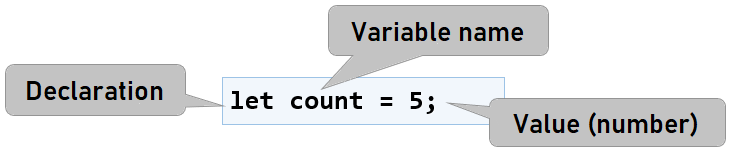After processing, the data is stored again in variables (i.e. somewhere in the memory set aside by our program).

## Data Types and Variables

In programming each variable stores a certain value of a particular type. For example, data types can be a number, string (text), boolean type, data, list, etc. Here are some examples of data types and values for them:

• number - type of number: 1, 42, -5, 3.14, NaN, …
• string - type of text (string): 'Hello', "Hi", 'Beer', …
• boolean - boolean type: true, false
• Date - date: Tue Jul 04 2017, ……

JavaScript language has three keywords for declaring a variable - var, const, and let. The main difference between let and var is in the scope of the variable. We use const when we are sure that what we assign to the variable will not change. A little further in the book, we will find out more details about the range of variables but for now, we will use the word let to declare a new variable.

For printing text, number, or another result on the screen, it's necessary to call the built-in method console.log(). With it we can print both the value of a variable and directly text or number:

console.log(42); // prints number

console.log('Hello!'); // prints string

let msg = 'Hello, JavaScript!';
console.log(msg); // prints a value of variable


## Reading a User Input as an Integer:

For reading a user input as a number is necessary to define an argument of our function:

function sum([arg1, arg2]) {
let a = parseInt(arg1);
let b = parseInt(arg2);
...
}


Let's note that the arguments arg1 and arg2 can be a different data type than the one we want. That's why it's necessary to convert them into a suitable one. If it's not done for the program each number will be just a string with which we can't do operations arithmetic operations.

### Example: Calculating a Square Area with Length of a Side a

For example, let's look at the following function which reads an integer from the console, multiplies it by itself (squares it), and prints the result from the multiplication. That's how we can calculate square area by side length:

function calculateSquareArea([arg1]) {
let a = parseInt(arg1);
let area = a * a;
console.log('Square area = ' + area);
}


If we call our function with parameter 3 - calculateSquareArea() the result will be - Square area = 9. Here's how our code looks like in action in the web browser's JavaScript console: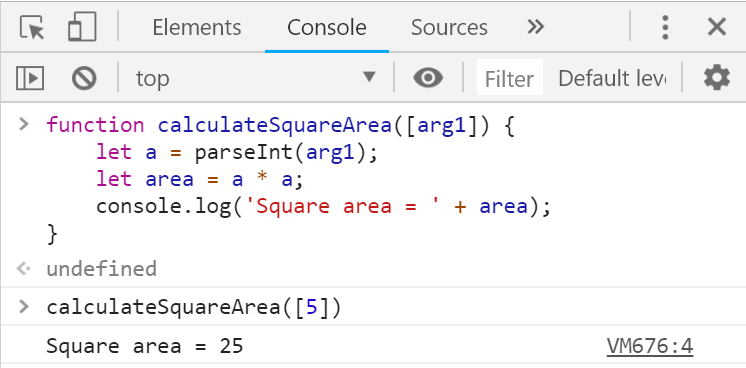If we try to write a wrong number, for example, "hello", we will get an error message during runtime (exception). This is normal. Later on, we will find out how we can catch these kinds of errors and make the user enter a number again.

#### How Does the Example Work?

On the first line with function calculateSquareArea([arg1]) { we define our function by giving it a name and setting arguments that it needs. In our case, we have one argument which is the side of the square.

On the next line with let a = parseInt(arg1); we get the argument of the function arg1 and convert it to an integer with the method parseInt(arg1);. The result is saved in variable a.

Note: If arg1 contains a floating-point number, that will be rounded to an integer. Converting a floating number to an integer is performed by removing all digits after the decimal point. Example: parseInt(2.3) = 2, parseInt(3.8) = 3

The next command let area = a * a; is saved in a new variable named area - the result of the multiplication a by a.

The next command console.log('Square area = ' + area); prints the specified text by placing the calculated face of the square, which we have saved in the variable area next to it.

The above program can be simplified a bit, like this:

function calculateSquareArea([a]) {
let area = a * a;
console.log('Square area = ' + area);
}


The above code will work correctly because when multiplied, the variable a will be converted to a number. When the input is only a single number, the parentheses [] can also be skipped, like this:

function calculateSquareArea(a) {
let area = a * a;
console.log('Square area = ' + area);
}


The code can be compact even more, like this:

function calculateSquareArea(a) {
console.log('Square area = ' + a * a);
}


#### Testing in the Judge system

Test your solution here: https://judge.softuni.org/Contests/Practice/Index/927#0. Try all four solutions to the problem.

To read user input as floating-point number it's necessary again to define an argument to our function. The syntax is similar to reading an integer, but here we have to use the function parseFloat(...):

function sum([arg1, arg2]) {
let a = parseFloat(arg1);
let b = parseFloat(arg2);
...
}


### Example: Converting Inches into Centimeters

Let's write a function that reads a floating-point number in inches and converts it to centimeters::

function convertInchesToCentimeters([arg1]) {
let inches = parseFloat(arg1);
let centimeters = inches * 2.54;
console.log('Centimeters = ' + centimeters);
}


Let's call the function and make sure that when passing a value in inches, we get the correct result in centimeters:

convertInchesToCentimeters(); // Centimeters = 12.7


#### Testing in the Judge System

Same with other data types, to read a string it's necessary to define an argument to our function and after that to assign it to a variable:

function print([arg1]) {
let text = arg1;
...
}


### Example: Greeting by Name

Let's write a program that asks the user for their name and salutes them with the text "Hello, (name)".

function sayHello([arg1]) {
let name = arg1;
console.log(Hello, ${name}!); }  In this case, the expression ${name} will be replaced with the value of the variable name. If we call the function with the name "Ivan", that will be the result:

sayHello(['Ivan']); // Hello, Ivan!


## Concatenating Text and Numbers

When printing text, numbers and other data we can concatenate them by using templates variable = ${variable} . In programming these templates are called placeholders. Pay attention: We need to use italicized apostrophes  (backticks) instead of normal quotes to recognize the template: function printInfo([firstName, lastName, age, town]) { console.log(You are${firstName} ${lastName}, a${age}-years old person from ${town}.); }  We call the function with test parameters again and make sure that it works: printInfo(['Ivan', 'Ivanov', 20, 'Sofia']);  Except for variables, we can make simple calculations in the templates. The same variable can be used as a template more than once. Here's an example: let a = 1; console.log(${a} + ${a} =${a + a});


The result is:

1 + 1 = 2


## Arithmetic Operations

Let's examine the basic arithmetic operations in programming.

### Summing Numbers (operator +)

We can sum numbers using the operator +:

let a = 5;
let b = 7;
let sum = a + b; // the result is 12


### Subtracting Numbers (operator -)

Subtracting numbers is done using the - operator:

function substractNumbers([arg1, arg2]) {
let a = parseInt(a);
let b = parseInt(b);
let result = a - b;
console.log(result);
}


Let we check the result of the execution of this program (with numbers 10 and 3):

substractNumbers([10, 3]); // 7


### Multiplying Numbers (operator *)

For multiplication of numbers we use the * operator:

let a = 5;
let b = 7;
let product = a * b; // 35


### Dividing Numbers (operator /)

Dividing numbers is done using the / operator.

Note: Float numbers divided by 0 do not cause an exception and the result is +/- infinity or the special value Infinity.

Here are a few examples with the division operator:

console.log(10 / 2.5); // Result: 4
console.log(10 / 4);   // Result: 2.5
console.log(10 / 6);   // Result: 1.6666666666666667

console.log(a / 0);   // Result: Infinity
console.log(-a / 0);  // Result: -Infinity
console.log(0 / 0);   // Result: NaN (Not a Number), i.e. the result
// The operation  hasn't a valid numeric value


## Concatenating Text and Numbers

Besides summing up numbers, the operator + is also used for joining pieces of text (concatenation of two strings one after another). In programming, joining two pieces of text is called "concatenation". Here is how we can concatenate a text with a number by the + operator:

let firstName = "Maria";
let lastName = "Ivanova";
let age = 19;
let str = firstName + " " + lastName + " @ " + age;
console.log(str);  // Maria Ivanova @ 19


Here is another example:

let a = 1.5;
let b = 2.5;
let sum = "The sum is: " + a + b;
console.log(sum);  // The sum is: 1.52.5


Do you notice anything strange? Maybe you expected the numbers a and b to sum? The concatenation works from left to right and the above result is correct. If we want to sum the numbers, we will have to use brackets to change the order of operations:

let a = 1.5;
let b = 2.5;
let sum = "The sum is: " + (a + b);
console.log(sum);  // The sum is: 4


## Numerical Expressions

In programming, we can calculate numerical expressions, for example:

let expr = (3 + 5) * (4 – 2);


The standard rule for priorities of arithmetic operations is applied: multiplying and dividing are always done before adding and subtracting. In the case of an expression in brackets, it is calculated first but we already know all of that from the school math.

### Example: Calculating Trapezoid Area

Let's write a program that inputs the lengths of the two bases of a trapezoid and its height (one floating-point number per line) and calculates the trapezoid area by the standard math formula:

function printTrapezoidArea([arg1, arg2, arg3]) {
let b1 = parseFloat(arg1);
let b2 = parseFloat(arg2);
let h = parseFloat(arg3);
let area = (b1 + b2) * h / 2;
console.log("Trapezoid area = " + area);
}


Because we want our function to work with both integers and floating numbers, we use parseFloat(). If we start the function and enter values for sides: 3, 4, and 5, we will obtain the following result::

printTrapezoidArea([3, 4, 5]); // Trapezoid area = 17.5


## Rounding Numbers

Sometimes when we work with floating numbers, it's necessary to bring them to integers. This bringing is named rounding. The programming language JavaScript provides us with several methods for rounding numbers:

• Math.ceil(…) - rounding up to next (greater) integer:
let up = Math.ceil(45.15); // up = 46

• Math.floor(…) - rounding down to previous (less) integer:

let down = Math.floor(45.67);    // down = 45

• Math.trunc(…) - cutting the decimal places:

let trunc = Math.trunc(45.67); // trunc = 45

• Math.round(…) - rounding is done as a basic rule for rounding numbers - if the decimal part is less than 5, rounding is to the previous number and if it's greater than 5 - to the next:

Math.round(5.439); // 5
Math.round(5.539); // 6

• .toFixed([number of characters after the decimal point]) - rounding to the closest number:

(123.456).toFixed(2);     // 123.46
(123).toFixed(2);         // 123.00
(123.456).toFixed(0);     // 123
(123.512).toFixed(0);     // 124


### Example: Circle Area and Perimeter

Let's write a function that receives an input of the radius r of a circle and calculates the area and the perimeter of the circle.

Formulas:

• Area = π * r * r
• Perimeter = 2 * π * r
• π ≈ 3.14159265358979323846…
function calculateCircleAreaAndPerimeter([arg1]) {
let r = parseInt(arg1);
console.log("Area = " + Math.PI * r * r);
// Math.PI - Built-in JavaScript constant for the value of the number π
console.log("Perimeter = " + 2 * Math.PI * r);
}


Let's call the function with radius r = 10:

calculateCircleAreaAndPerimeter()


The result is: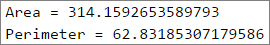### Example: 2D Rectangle Area

The rectangle is given with the coordinates of two of its opposite angles. Calculate its area and perimeter :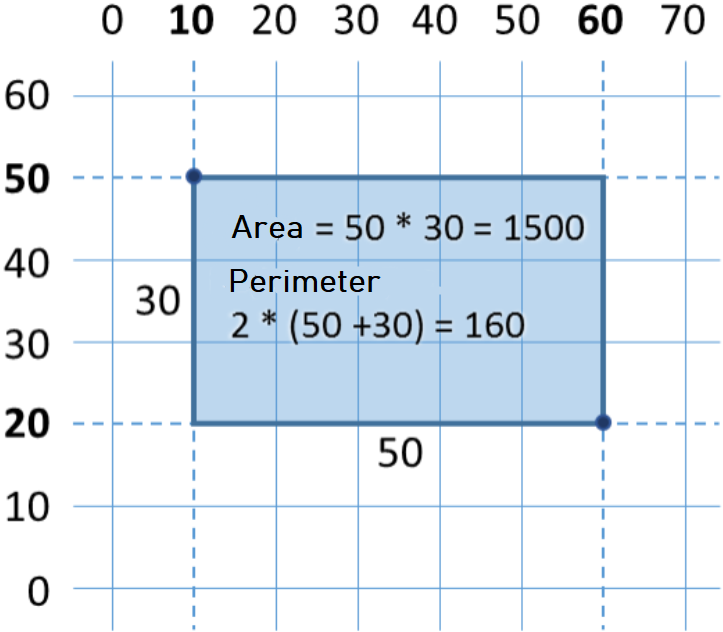In this problem, we have to consider that if we subtract the smaller x from the bigger x , we will obtain the length of the rectangle. Identically, if we subtract the smaller y from the bigger y, , we will obtain the height of the rectangle. What is left is to multiply both sides. Here is an example of an implementation of the described logic:

function calculateRectangleArea([arg1, arg2, arg3, arg4]) {
let x1 = parseFloat(arg1);
let y1 = parseFloat(arg2);
let x2 = parseFloat(arg3);
let y2 = parseFloat(arg4);

// Calculating the sides of the rectangle:
let width = Math.max(x1, x2) - Math.min(x1, x2);
let height = Math.max(y1, y2) - Math.min(y1, y2);

console.log(width * height);
console.log(2 * (width + height));
}


We use the method Math.max(x1, x2) to find the higher value from x1 and x2 and identically Math.min(y1, y2) to find the lower of both values.

Let we call the function with testing values from the coordinate system:

calculateRectangleArea([60, 20, 10, 50]); // 1500
// 160


## What have we learned from this chapter?

Let's summarize what we learned from this chapter of the book:

• Reading an user input: function sum([number1, number2]).
• Converting to number: let num = parseInt(arg1), let num = parseFloat(arg1).
• Aritmetic operations and using the relevant aritmetic operators [+, -, *, /, ()]: let sum = 5 + 3.
• Print text by using concatenation: console.log(3 + 5 = \${3 + 5}).
• The different types of rounding numbers: Math.ceil(), Math.trunc(), Math.floor() and .toFixed()

## Exercises: Simple Calculations

Let's strengthen the knowledge gained throughout this chapter with a few more exercises.

### Empty JS File for our Solution of the Problem in Visual Studio Code

We start by creating an empty JS file in Visual Studio Code. In the current practical exercise we'll create and add a new JS file for each task, to organize the solutions of the tasks from the exercises:

We start Visual Studio Code and create a New File: [File] -> [New File]: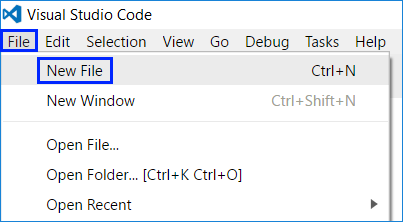Save the file from [File] -> [Save] or by keyboard shortcut [Ctrl + S]: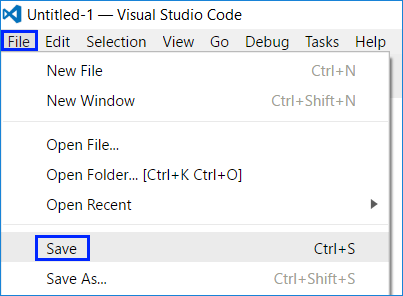Enter a significant name and expansion .js to our file, then press the button [Save]: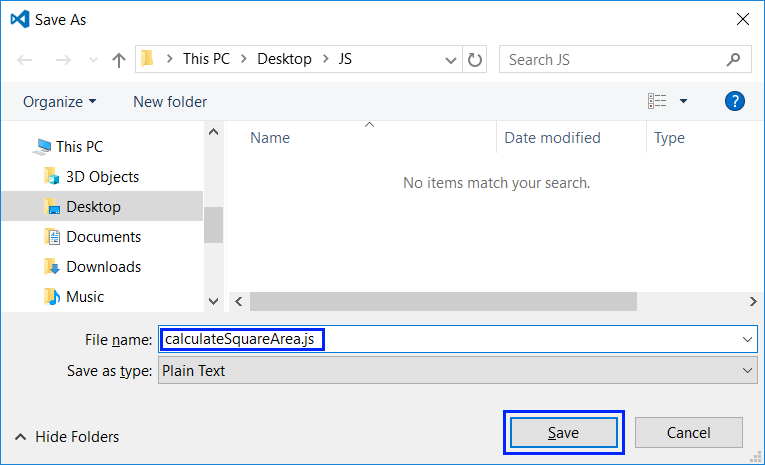### Problem: Calculating Square Area

The first exercise from this topic is the following: write a function that receives an integer a and calculates the area of a square with side a. The problem is trivially easy: inputs a number as an argument of the function, multiply it by itself, and prints a result on the console.

#### Hints and Guidelines

We have a correctly named empty file. What remains is to write the code for solving the problem. For this purpose, we write the following code: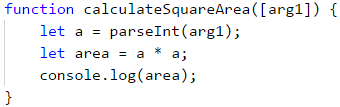The code defines a function calculateSquareArea(), which receives one argument arg1. Since the argument is expected to be an integer, convert arguments with the method parseInt() and then calculate the area: area = a * a. At the end print the value of the variable area. For testing, it's necessary to call the function in the same file with a random parameter and then to start the program by pressing [Ctrl + F5]: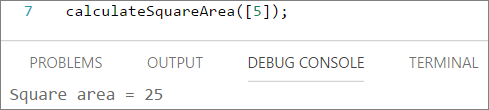#### Testing in the Judge System

You must get 100 points (completely correct solution):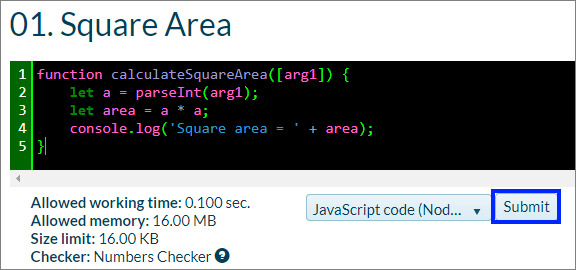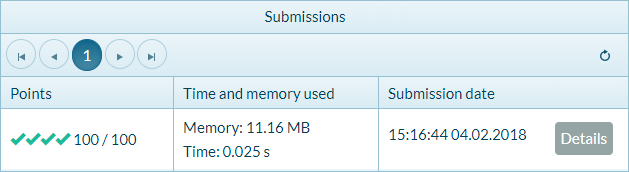### Problem: From Inches to Centimeters

Write a function that accepts a number (not necessarily an integer) and converts the number from inches to centimeters. For this purpose, it multiplies the inches by 2.54 (because 1 inch = 2.54 centimeters).

#### Hints and Guidelines

First, we create a new file in the folder with the other solutions - in Visual Studio Code we choose [File] -> [New file]. We save the file with the name convertInchesToCentimeters.js and press the button [Save]. After that we have to write the program code: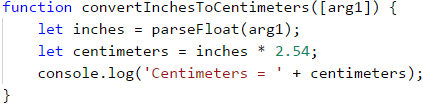We call the function with parameter 2 and start the problem with [Ctrl + F5]: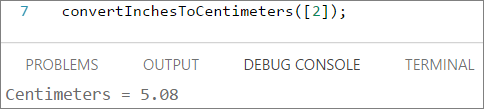Let's test it with floating numbers, for example, 4.5: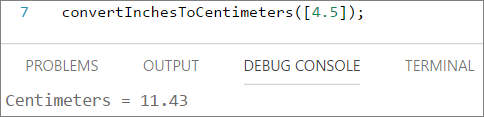#### Testing in the Judge System

The solution should be taken as completely correct: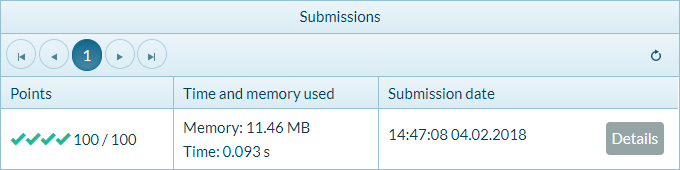### Problem: Greeting by Name

Write a function, which takes as an argument a human name and prints Hello, <name>!, where <name> is the name entered earlier.

#### Hints and Guidelines

Again we create a new file in the folder with the other solutions and we save the file with the name sayHello.js. After that, we have to write the program code. If you find it difficult, you can use the sample code below: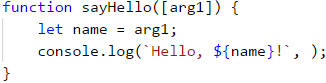We call the function with an example parameter and start the program with [Ctrl+F5], to test if it works properly: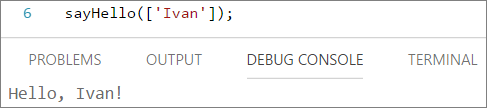### Problem: Concatenating Text and Numbers

Write a function that receives as an argument the name, surname, age and city and print a message of the following type: You are <firstName> <lastName>, a <age>-years old person from <town>.

#### Hints and Guidelines

Similarly, we create a new file and name it printInfo.js. which prints the message described in the condition of the task, is purposefully blurred for you to think of a way to finish it yourself: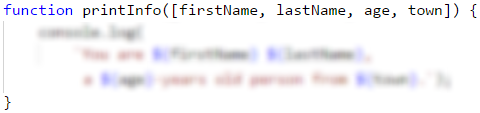The solution should be tested locally by calling the function with sample values and starting the program with [Ctrl+F5].

### Problem: Trapezoid Area

Write a function that receives three arguments b1, b2, and h and calculate the trapezoid area with bases b1 and b2 and height h. The formula for trapezoid area is (b1 + b2) * h / 2.

The figure below shows a trapezoid with bases 8 and 13 and height 7. It has an area (8 + 13) * 7 / 2 = 73.5.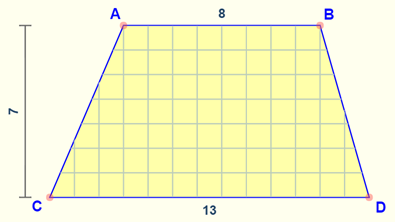#### Hints and Guidelines

We should add a new file in Visual Studio Code again with the name calculateTrapezoidArea.js and write a code that reads the inputs from the function's arguments, calculates the trapezoid area, and prints it. The code in the picture is purposely blurred, for you to give it a thought and finish it yourself: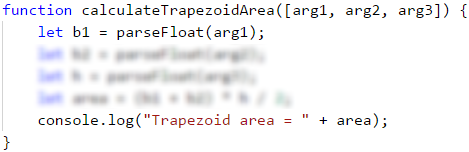Test your solution locally by calling the function and starting the program with [Ctrl+F5].

### Problem: Circle Perimeter and Area

Write a function, that receives an argument number r and calculates and prints the area and perimeter of a circle with radius r.

#### Sample Input and Output

Input Output
3 Area = 28.2743338823081
Perimeter = 18.8495559215388
4.5 Area = 63.6172512351933
Perimeter = 28.2743338823081

#### Hints and Guidelines

For the calculations you may use the following formulas:

• Area = Math.PI * r * r.
• Perimeter = 2 * Math.PI * r.

### Problem: Rectangle Area

A Rectangle is defined by the coordinates of both of its opposite corners (x1, y1) – (x2, y2). Calculate its area and perimeter. The input is taken as a function argument. The numbers x1, y1, x2, and y2 are given one per line. The output is printed on the console and it has to contain two lines, each with one number – the area and the perimeter.Input Output
60
20
10
50
1500
160
30
40
70
-10
2000
180
600.25
500.75
100.50
-200.5
350449.6875
2402

### Problem: Triangle Area

Write a function that receives arguments which are a side and a height of a triangle and calculates its area. Use the formula for triangle area: area = a * h / 2. Round the result to 2 digits after the decimal point using area.toFixed(2).

#### Sample Input and Output

Input Output
20
30
Triangle area = 300
15
35
Triangle area = 262.5
7.75
8.45
Triangle area = 32.74
1.23456
4.56789
Triangle area = 2.82

### Problem: Converter – from °C Degrees to °F Degrees

Write a function that reads degrees on the Celsius scale (°C) and converts them to degrees on the Fahrenheit scale (°F). Look on the Internet for a proper formula to do the calculations. Round the result to 2 digits after the decimal point. Here are a few examples:

Input Output
25 77
0 32
-5.5 22.1
32.3 90.14

### Problem: Converter - Radians to Degrees

Write a function that reads an angle in radians (rad) and converts it in degrees) (deg). Look for a proper formula on the Internet. The number π in JavaScript programs is available through Math.PI. Round the result to the nearest integer using the Math.round(…) method.

Input Output
3.1416 180
6.2832 360
0.7854 45
0.5236 30

### Problem: Converter - USD to BGN

Write a function for conversion of US dollars (USD) into Bulgarian levs (BGN). Round the result to 2 digits after the decimal point. Use a fixed rate between a dollar and lev: 1 USD = 1.79549 BGN.

Input Output
20 35.91 BGN
100 179.55 BGN
12.5 22.44 BGN

### Problem: * Currency Converter

Write a function for the conversion of money from one currency into another. It has to support the following currencies: BGN, USD, EUR, GBP. Use the following fixed currency rates:

Rate USD EUR GBP
1 BGN 1.79549 1.95583 2.53405

The input is a sum for conversion, input currency, and output currency. The output is one number – the converted value of the above currency rates rounded 2 digits after the decimal point.

Input Output
20
USD
BGN
35.91 BGN
100
BGN
EUR
51.13 EUR
12.35
EUR
GBP
9.53 GBP
150.35
USD
EUR
138.02 EUR

### Problem: ** Date Calculations – 1000 Days on the Earth

Write a function that reads a birth date in format dd-MM-yyyy and calculates the date on which 1000 days are turned since this birth date and prints it in the same format.

#### Sample Input and Output

Input Output
1995-02-25 20-11-1997
2003-11-07 02-08-2006
2002-12-30 24-09-2005
2012-01-01 26-09-2014
1980-06-14 10-03-1983

#### Hints and Guidelines

• Look for information about the data type Date in JavaScript and in particular look at the methods setDate(...), getDate(), getMonth() and getYear(). With their help, you can solve the problem without the need to calculate days, months, and leap years.
• Don't print anything additional on the console except for the wanted date!

## Graphic Applications with Numerical Expressions

To exercise working with variables and calculations with operators and numerical expressions, we will make something interesting: we will develop a web application with a graphical user interface. In it, we will use calculations with floating-point numbers.

### Web Application: *** Converter - BGN to EUR!

Create a web application that calculates the value in Euro (EUR) of the monetary amount given in Bulgarian levs (BGN). By changing the amount in BGN, the amount in EUR has to be recalculated automatically. Use the fixed-rate BGN / Euro: 1.95583.Similarly, as in the first chapter ("First Steps in Programming"), we will use the languages JavaScript, HTML, and CSS for our application.

1. The first step is to create a folder in which we will store all the files that are needed for our application.
2. Then we need to create an HTML file in the folder: index.html
<!DOCTYPE html>

<html>
<meta charset="utf-8">
<title>BGN to EUR Converter</title>
<body>
<form class="content-form">
<h2 class="title">BGN to EUR Converter</h2>
<section class="items">
<label for="bgn" class="currency">
<span class="item-currency">BGN: </span>
<input class="currency-value" type="number" id="bgn" value="0" />
</label>
<label for="euro" class="currency">
<span class="item-currency">EUR: </span>
<input class="currency-value" type="text" id="euro" readonly />
</label>
<input class="primary-btn" type="button" value="Convert!" />
</section>
</form>
</body>
</html>


Note that each HTML page must have a specific structure. For example, always the main code we write is in the tag <body>, and always the title of the page is in the tag <title>.

1. We have the structure of the page, it remains to add a JavaScript file with the logic itself. We create a new file and name it converter.js
function eurConverter() {
let bgn = document.getElementById("bgn").value;
let eur = (bgn / 1.95583).toFixed(2);
document.getElementById("euro").value = eur;
}

1. Once we have the logic of the application, we need to find a way to tell where to use it. To do this we need to make 2 changes to the existing index.html file:

First, we add the following line just below the title tag, through which the connection between the files is made index.html and converter.js:

 <script src="converter.js" type="text/javascript"></script>


And second, we find and replace the input field with the type button with the following code. In this way we set when clicking on the button [Convert!] to call the function eurConverter():

<input class="primary-btn" type="button" onclick="eurConverter()" value="Convert!" />


If we start the file index.html from the folder, we should have a working application that converts from BGN to EUR: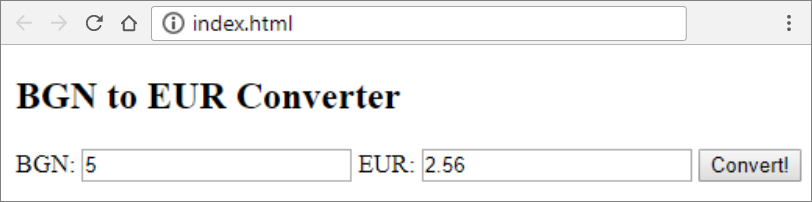Let's make it more beautiful.

1. We create a new file with extension *.css and name index. CSS is used to stylize the elements in HTML. We open the file index.html and add this line in the tag <head>:
<link rel="stylesheet" href="index.css" type="text/css" />


In the file index.css we write the following code (we define styles for the individual elements of the HTML format):

body {
font-family: 'Lato', sans-serif;
color: #FFFFFF;
}

.content-form {
width: 50%;
margin: 5% auto;
background: #234465;
box-shadow: 5px 5px 10px #808080, 5px 5px 10px #6793c1 inset;
}

.currency-value {
border: none;
}

.title {
text-align: center;
}

.item-currency {
font-weight: 700;
}

.currency {
margin: auto;
}

.items {
display: flex;
flex-direction: column;
justify-content: flex-start;
}

.primary-btn {
margin: auto;
border: none;
background-color: #ffa000;
color: #FFFFFF;
font-weight: 700;
}

1. Start the file index.html: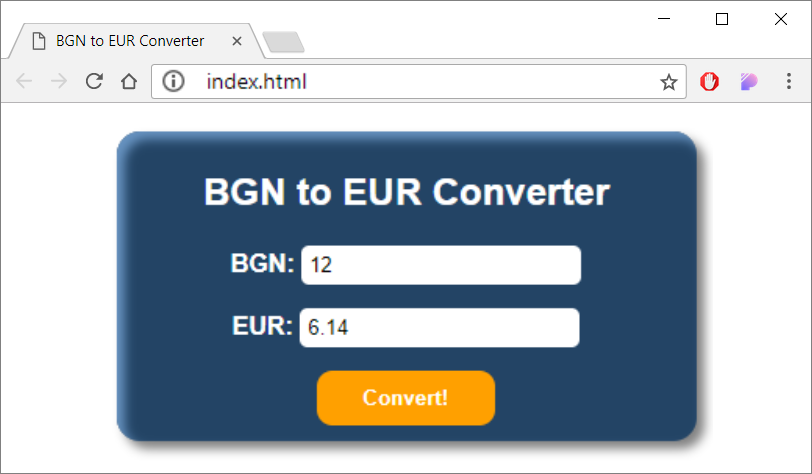### Web Application: *** Catch the Mouse!

Upon moving the mouse cursor onto the button, it moves to a random position. This way it creates the impression that the image runs from the mouse and it is hard to catch“. When the image gets “caught”, a congratulations message is shown.

Hint: Write an Event Handler mouseover and move the image to a random position. Use the random numbers generator Math.random(). The position of the image is set from the property style.position. To "catch the mouse" onclick to "catch the mouse".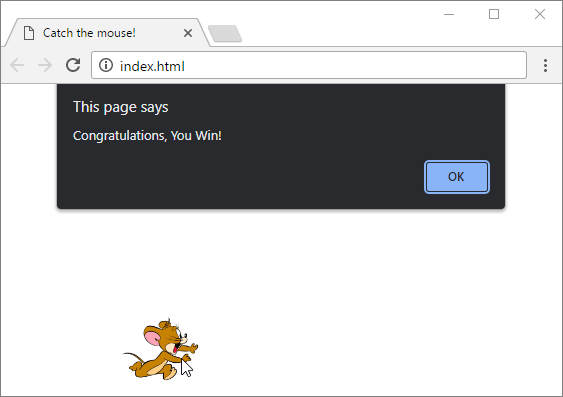1. We create a new folder catch-the-mouse in which we will save the files for the application.
2. We create two files in the folder: index.html and app.js. The folder structure must look like this: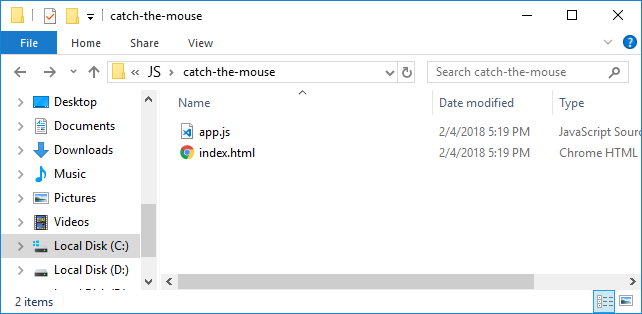The file index.html must look like this:

<!DOCTYPE html>

<html>
<meta charset="utf-8" />
<title>Catch the mouse!</title>
<script src="app.js" type="text/javascript"></script>
<body>
<img id="image" style="position:absolute;top:30%;left:30%; width:10%; height:auto; " src="images/mouse.jpg" />
</body>
</html>


The file app.js must look like this:

function chaseMouse() {
let img = document.getElementById("image");
img.style.position = "absolute";
img.style.left = (Math.random() * 300) + "px";
img.style.top = (Math.random() * 300) + "px";
}

function catchMouse() {
}

1. We find an image from the internet and add it by naming it mouse.jpg`.

Test the application by opening the project folder in explorer and launching the file index.html: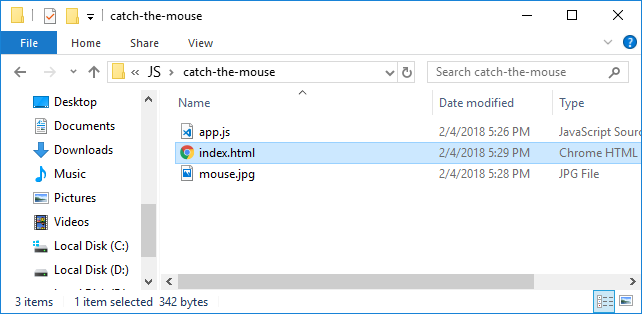1. Done the application.

If you have any difficulties, ask in the Softuni Forum: https://softuni.bg/forum.